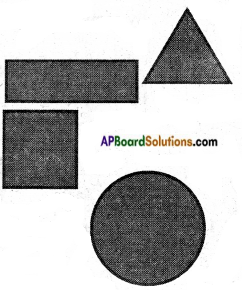# AP Board 6th Class Maths Notes Chapter 11 Perimeter and Area

Students can go through AP Board 6th Class Maths Notes Chapter 11 Perimeter and Area to understand and remember the concepts easily.

## AP State Board Syllabus 6th Class Maths Notes Chapter 11 Perimeter and Area

→ Perimeter: The perimeter of a polygon is sum of all its sides.
The perimeter of an equilateral triangle is P = 3 × side
The perimeter of a rectangle P = 2 (length + breadth)
And its area A = length × breadth A = l × b
The perimeter of a square is P = 4 × side And its area A = side × side (or)
A = s × s The circumference of a circle C = 2πr where r is the radius of the circle.→ Area: The region occupied by a plane figure is called its area.
To find the area of a complex figure, we divide the given shape into the combination of rectangles, squares and triangles where ever necessary.